Stat 330 Assignment 9 Partial Solutions

1. Chapter 11 Q4: I ran SAS and, after editing, I got the following output:
```                        General Linear Models Procedure
Dependent Variable: COVER
Sum of            Mean
Source             DF          Squares          Square   F Value     Pr > F
PAINT               3     296.25000000     98.75000000     10.97     0.0075
ROLLER              2       4.66666667      2.33333333      0.26     0.7798
Error               6      54.00000000      9.00000000
Corrected Total    11     354.91666667

R-Square             C.V.        Root MSE           COVER Mean
0.847852         6.581353       3.0000000            45.583333

Tukey's Studentized Range (HSD) Test for variable: COVER
Alpha= 0.05  Confidence= 0.95  df= 6  MSE= 9
Critical Value of Studentized Range= 4.896
Minimum Significant Difference= 8.4794
Comparisons significant at the 0.05 level are indicated by '***'.

Simultaneous            Simultaneous
Lower    Difference     Upper
PAINT        Confidence    Between   Confidence
Comparison        Limit       Means       Limit

1    - 2          -0.479       8.000      16.479
1    - 3           3.521      12.000      20.479   ***
1    - 4           3.854      12.333      20.813   ***
2    - 3          -4.479       4.000      12.479
2    - 4          -4.146       4.333      12.813
3    - 4          -8.146       0.333       8.813```
I see a clear effect of paint brand but no visible effect of roller brand. Brand 1 is better than 3 or 4 but not definitely better than 2. However, even that difference is nearly significant.
2. Chapter 11 Q 6: We find MSA=11.7/2=5.85, MSB=113.5/4=28.375 and MSE = 25.6/8=3.2. The F statistic for the hypothesis of no difference between assessors is 5.85/3.2 =1.83 which is not significant at the 5% level (the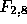critical value is 4.46. The design is chosen to make sure that variations between values for different assessors are not due to house value differences. A design in which the different assessors assessed different houses would be much less sensitive to small differences between the assessors because the variation in value from house to house is large compared to the likely size of the variation from assessor to assessor. Note that the effect due to houses is large and statistically significant but that no one would test this hypothesis since we all know different houses have different values.
3. Q 14:
```Source   SS     df   MS            F      P
A      30763    2   15381.5     3.79     0.037
B      34185.6  3   11728.5     2.81     0.061
A*B    43581.2  6    7263.5     1.79     0.144
Error  97436.8  24   4059.9
Total 205966.6  35```
The interactions are not significant. The main effect of Factor A is marginally significant while that of B is marginally not so. Generally it seems likely that curing time has an effect on compressive strength and that Factor B might do too. The Tukey intervals for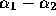,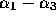and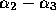are all estimate plus or minus (2.92)(63.7)/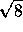. (The number 63.7 is just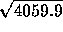.) NOTE: this is a typical exam type question.
4. Q16: I got the following from SAS. It shows no real evidence of interactions (P=0.25) and significant main effects of both formula and speed. It shows that the speed 70 gives a significantly lower yield than either the lower or higher speed. To get estimates of the main effects you need to average the columns and subtract the grand mean or average the top 9 numbers and bottom 9 numbers in the table and then subtract the grand mean. I did not produce the probability plot though I think you know how to do so with SAS.
```                        General Linear Models Procedure
Dependent Variable: YIELD
Sum of            Mean
Source             DF          Squares          Square   F Value     Pr > F
FORMULA             1     2253.4422222    2253.4422222    376.27     0.0001
SPEED               2      230.8144444     115.4072222     19.27     0.0002
FORMULA*SPEED       2       18.5811111       9.2905556      1.55     0.2516
Error              12       71.8666667       5.9888889
Corrected Total    17     2574.7044444

R-Square             C.V.        Root MSE           YIELD Mean
0.972087         1.391696       2.4472206            175.84444

Tukey's Studentized Range (HSD) Test for variable: YIELD
Alpha= 0.05  Confidence= 0.95  df= 12  MSE= 5.988889
Critical Value of Studentized Range= 3.773
Minimum Significant Difference= 3.7693
Comparisons significant at the 0.05 level are indicated by '***'.

Simultaneous            Simultaneous
Lower    Difference     Upper
SPEED        Confidence    Between   Confidence
Comparison        Limit       Means       Limit

80   - 60         -2.719       1.050       4.819
80   - 70          4.297       8.067      11.836   ***
70   - 60        -10.786      -7.017      -3.247   ***```
5. Q48: I used proc glm with the statement model smooth = method fabric to get the following output which shows a very clear effect of drying method. There is no need to look at the effect of fabric; as a blocking variable it would be surprising if it did not have an effect. Tukey's procedure shows that drying methods are divided into two groups: methods 1 and 3 giving significantly less smoothness than 2, 4 or 5. Note that I have rearranged the SAS output to match the form in the text.
```                        General Linear Models Procedure
Dependent Variable: SMOOTH
Sum of            Mean
Source             DF          Squares          Square   F Value     Pr > F
FABRIC              8       9.69600000      1.21200000     11.89     0.0001
METHOD              4      14.96222222      3.74055556     36.70     0.0001
Error              32       3.26177778      0.10193056
Corrected Total    44      27.92000000

R-Square             C.V.        Root MSE          SMOOTH Mean
0.883174         12.94320       0.3192657            2.4666667

Tukey's Studentized Range (HSD) Test for variable: SMOOTH
Alpha= 0.05  df= 32  MSE= 0.101931
Critical Value of Studentized Range= 4.086
Minimum Significant Difference= 0.4349
Means with the same letter are not significantly different.
Tukey Grouping              Mean      N  METHOD

A            3.3556      9  1
A
A            2.9556      9  3

B            2.0222      9  4
B
B            2.0111      9  5
B
B            1.9889      9  2```
6. Q 50: Most students will simply have done a two way anova on this data set and found no significant effect of Sowing Rate. However, the very high variability within plot 1 and low variability within plots 3 and 4 suggests that the assumption of constant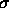is probably wrong. There is a test, called Tukey's one degree of freedom test for non-additivity which would have suggested a transformation is needed. I analyzed the logarithms of the clover accumulations and concluded that there probably is a difference. First the SAS code:
```options pagesize=60 linesize=80;
data Q50;
infile 'q50.dat';
input plot rate clover;
logcl=log(clover);
proc glm  data=Q50;
class plot rate;
model logcl = plot rate;
means rate / tukey cldiff alpha=0.05;
run;```
and some of the output:
```                        General Linear Models Procedure
Dependent Variable: LOGCL
Sum of      Mean
Source             DF      Squares     Square   F Value     Pr > F
Model               6      24.064      4.0107     19.91     0.0001
Error               9       1.813      0.2014
Corrected Total    15      25.877
Root MSE           LOGCL Mean
0.4488151            6.1196277

Source        DF   Type I SS  Mean Square   F Value     Pr > F
PLOT          3      16.740      5.58        27.70     0.0001
RATE          3       7.324      2.44        12.12     0.0016
Tukey's Studentized Range (HSD) Test for variable: LOGCL
Alpha= 0.05  Confidence= 0.95  df= 9  MSE= 0.201435
Critical Value of Studentized Range= 4.415
Minimum Significant Difference= 0.9907
Simultaneous            Simultaneous
Lower    Difference     Upper
RATE        Confidence    Between   Confidence
Comparison        Limit       Means       Limit

13.5 - 10.2      -1.0257     -0.0350      0.9558
13.5 - 6.6       -0.3236      0.6671      1.6579
13.5 - 3.6        0.6426      1.6333      2.6241   ***
10.2 - 6.6       -0.2886      0.7021      1.6929
10.2 - 3.6        0.6776      1.6683      2.6590   ***
6.6  - 3.6       -0.0246      0.9662      1.9569```
I have rearranged things. Note that the procedure analyzes means of the logarithm not of the original variable. The conclusions are that there is an effect to Sowing Rate and that the lowest level is definitely worse than either of the two highest levels at producing clover. To get the same analysis on the original scale you drop mention of logcl and put clover in the model statement.

Richard Lockhart
Wed Apr 1 15:31:24 PST 1998